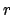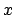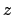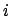Next: menu Up: Tcl Text Commands Previous: material   Contents   Index

## measure

The measure command supplies several algorithms for analyzing molecular structures. In the following options, selection refers to an atom selection, as returned by the atomselect command described in section 8.3.2. The optional weight must be either none, an atom selection keyword such as mass, or a list of values, one for each atom in the selection, to be used as weights. If weight is missing or is none, then all weights are taken to be 1. When an atom selection keyword is used, the weights are taken from selection1.

• avpos selection [first first] [last last] [step step]: Returns the average position of each of the selected atoms, for the selected frames. If no first, last, or step values are provided the calculation will be done for all frames.

• center selection [weight weight]: Returns the geometric center of atoms in selection using the given weight.

• contacts cutoff selection1 [selection2]: Find all atoms in selection1 that are within cutoff of any atom in selection2 and not bonded to it. If selection2 is omitted, it is taken to be the same as selection1. selection2 and selection1 can either be from the same of from different molecules. Returns two lists of atom indices, the first containing the first index of each pair (taken from selection1) and the second containing the second index (taken from selection2). Note that the index is the global index of the atom with respect to its parent molecule, as opposed to the index within the given atom selection that contains it.

• fit selection1 selection2 [weight weight]: Returns a 4x4 transformation matrix which, when applied to the atoms in selection1, minimizes the weighted RMSD between selection1 and selection2. See section 11.4.2 for more on RMSD alignment.

• gofr selection1 selection2 [delta value] [rmax value] [usepbc boolean] [selupdate boolean] [first value] [last value] [step value]: Calculates the atomic radial pair distribution functionand the number integralfor all pairs of atoms in the two selections. Both selections have to reference the same molecule and may be identical. In case one of the selections resolves to an empty list for a given time step, and empty array is added to the histograms. The command returns a list of five lists containing,,, the unnormalized histogram, and a list of frame counters containing currently 3 elements: total number of frames processed, the number of skipped frames and the number of frames handled with the orthogonal cell algorithm (Further algorithm and corresponding list entris will be added in the future). With the optional arguments delta (default 0.1) and rmax (default 10.0) one can set the resolution and the maximumvalue. With the usepbc flag processing of periodic boundary conditions can be turned on. With the selupdate flag enabled, both atom selections are updated as each frame is processed, allowing productive use of "within" selections. The size of the unitcell has to be stored in the trajectory file or has to be set manually for all frames with the molinfo command. The command uses by default only the current active frame for both selections. Using an explicite frame range via first, last, and step is recommended for most cases.

• hbonds cutoff angle selection1 [selection2]: Find all hydrogen bonds in the given selection(s), using simple geometric criteria. Donor and acceptor must be within the cutoff distance, and the angle formed by the donor, hydrogen, and acceptor must be less than angle from 180 degrees. Only non-hydrogen atoms are considered in either selection. If both selection1 and selection2 are given, the selection1 is considered the donor and selection2 is considered the acceptor. If only one selection is given, all non-hydrogen atoms in the selection are considered as both donors and acceptors. The two selections must be from the same molecule. The function returns three lists; each element in each list corresponds to one hydrogen bond. The first list contains the indices of the donors, the second contains the indices of the acceptors, and the third contains the index of the hydrogen atom in the hydrogen bond.

Known Issue: The output of hbonds cannot be considered 100% accurate if the donor and acceptor selection share a common set of atoms.

• inverse matrix: Returns the inverse of the given 4x4 matrix.

• minmax selection: Returns two vectors, the first containing the minimum,, andcoordinates of all atoms in selection, and the second containing the corresponding maxima.

• rgyr selection [weight weight]: Returns the radius of gyration of atoms in selection using the given weight. The radius of gyration is computed as(8.1)

whereis the position of theth atom andis the weighted center as computer by measure center.

• rmsf selection [first first] [last last] [step step]: Returns the root mean square position fluctuation for each selected atom in the selected frames. If no first, last, or step values are provided the calculation will be done for all frames.

• rmsd selection1 selection2 [weight weight]: Returns the root mean square distance between corresponding atoms in the two selections, weighted by the given weight. selection1 and selection2 must contain the same number of atoms (the selections may be from different molecules that have different numbers of atoms).

• sasa srad selection [-points varname] [-restrict restrictedsel] [-samples numsamples]: Returns the solvent-accessible surface area of atoms in the selection using the assigned radius for each atom, extending each radius by srad to find the points on a sphere that are exposed to solvent. If the restrictedsel selection is used, only solvent-accessible points near that selection will be considered. The restrict option can be used to prevent internal protein voids or pockets from affecting the surface area results. The points option can be used to see where the area contributions are coming from, and then the restrict flag can be used to eliminate any unwanted contributions after visualizing them. The varname parameter can be used to collect the points which are determined to be solvent-accessible.

• sumweights selection weight weights: Returns the sum of the list of weights (or data field to use for the weights) for all of the atoms in the selection.

• bond atom_list [options]: Returns the distance of the two specified atoms. The atoms are specified in form of a list of atom indexes. Unless you specify a certain molecule through 'molid molecule_number' these indices refer to the current top molecule. If the atoms are in different molecules you can use the form {{atomid1 [molid1]} {atomid2 [molid2]} ... } where you can set the molecule ID for the individual atoms. Note that measure bond does not care about the bond that are specified in a psf file or that are drawn in VMD it just returns the distance! Similar things are true for measure angle, dihed and imprp.
The following options can be specified:
• molid <molecule_number>: The default molecule to which an atom belongs unless a molecule number was explicitely specified for this atom in the atom list. Further, all frame specifications refer to this molecule. (Default is the current top molecule.)
• frame <frame>: By default the value for the current frame will be returned but a specific frame can be chosen through this option. One can also specify all or last instead of a frame number in order to get a list of values for all frames or just the last frame respectively.
• first <frame>: Use this option to specify the first frame of a frame range. (Default is the current frame.)
• last <frame>: Use this option to specify the last frame of a frame range. (Default is the last frame of the molecule).
In case you specified the molecule IDs in the atom list then all frames specifications will refer to the current top molecule unless a default molecule was set using the 'molid' option. Since the top molecule can be different from the molecules involved in the selected atoms, it is generally a good idea to specify a default molecule.

Here are a few examples of usage:
measure bond {3 5} - Returns the bond energy between atoms 3 and 5 of the current frame of the top molecule. measure bond {3 5} molid 1 frame all - Returns the bond energy between atoms 3 and 5 of molecule 1 for all frames.
measure bond {3 {5 1}} molid 0 first 7 - Returns the bond energy between atoms 3 of molecule 0 and atom 5 of molecule 1. The value is computed for all frames between the seventh and the last frame of molecule 0.

• angle atom_list [options]: Returns the angle spanned by three atoms. Same input format as the 'measure bond' command.

• dihed atom_list [options]: Returns the dihedral angle defined by four atoms. Same input format as the 'measure bond' command.

• imprp atom_list [options]: Returns the improper dihedral angle defined by four atoms. Same input format as the 'measure bond' command.

• energy energy_term atom_list [parameters] [options]: Returns the specified energy term for a given set of atoms. The energy term must be one of bond, angle, dihed, imprp, vdw or elect where vdw stands for 'van der Waals' and elect for electrostatic energy. The energy is computed based on the CHARMM force field functions, the given parameters and the current coordinates. All options for the measure bond command work for measure energy, too. Thus, you can for instance request energies for a range of frames of a trajectory. Also the format of the atom list is the same. The following parameters can be specified:
• k <value>: force constant for bond, angle, dihed and imprp energies in kcal/mol/Aor kcal/mol/radrespectively.
• x0 <value>: equilibrium value for bond length, angle, dihedral angles and improper dihedrals in Angstrom or degree.
• kub <value>: Urey-Bradley force constant for angles in kcal/mol/A.
• s0 <value>: Urey-Bradley equilibrium distance for angles in Angstrom.
• n <value>: dihedral periodicity.
• delta <value>: dihedral phase shift in degree (usually 0.0 or 180.0).
• rmin1 <value>: VDW equilibrium distance for atom 1 in Angstrom.
• rmin2 <value>: VDW equilibrium distance for atom 2 in Angstrom.
• eps1 <value>: VDW energy well depth (epsilon) for atom 1 in kcal/mol.
• eps2 <value>: VDW energy well depth (epsilon) for atom 2 in kcal/mol.
• q1 <value>: charge for atom 1.
• q2 <value>: charge for atom 2.
• cutoff <value>: nonbonded cutoff distance.
• switchdist <value>: nonbonded switching distance.
For all omitted parameters a default value of 0.0 is assumed. For the electrostatic energy the default charges are taken from the according atom based field of the molecule. If the cutoff is not set or zero then no cutoff function will be used.Next: menu Up: Tcl Text Commands Previous: material   Contents   Index
vmd@ks.uiuc.edu